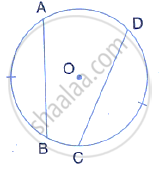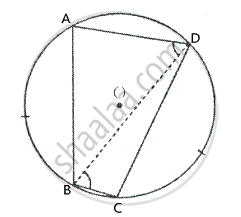Share

In the Given Figure, If Arc Ab = Arc Cd, Then Prove that the Quadrilateral Abcd is an Isosceles– Trapezium (O is the Centre of the Circle). - ICSE Class 10 - Mathematics

Question

In the given figure, if arc AB = arc CD, then prove that the quadrilateral ABCD is an isosceles– trapezium (O is the centre of the circle).SolutionGiven –
In the figure, O is the centre of a circle and arc AB = arc CD
To prove –
ABCD is an isosceles trapezium.
Construction – Join BD, AD and BC.
Proof – Since equal arcs subtends equal angles at the circumference of a circle.
∴ ∠ADB = ∠DBC               [∵ arc AB = arc CD]
But, these are alternate angles.